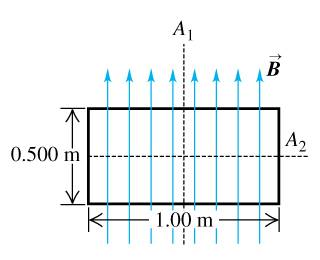# Rotation due to a magnetic field

• dpeagler

## Homework Statement

A uniform rectangular coil of total mass 270 and dimensions .5 m x .1 m is oriented perpendicular to a uniform 4.00-T magnetic field (the figure ). A current of 2.90 A is suddenly started in the coil.

## Homework Equations

angular acceleration = torgue / moment of inertia

## The Attempt at a Solution

I really don't know what to do on this one, simply because I don't know what to do for the moment of inertia term. I thought about breaking it up into the moment of inertia of the base and height sides, but I don't know how the mass is distributed so I can't do that.

If anyone can help that would be great.

Figure??